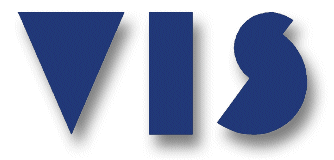## UNITS OF THE INTERNATIONAL SYSTEM

 The International System (abbreviated SI, for Systeme International, the French name for the system) was adopted in 1960 by the 11th General Conference on Weights and Measures.  An expanded and modified version of the metric system, the International System, addresses the need of modern science for additional and more accurate units of measurement.  The key features of the International System are decimalization, system of prefixes and a standard defined in terms of an invariable physical measure.### BASE UNITS

 The International System has base units from which all others in the system are derived.  The standards for the base units, except for the kilogram, are defined by unchanging and reproducible physical occurrences.  For example, the meter is defined as the distance traveled by light in a vacuum in 1/299,792,458 of a second.  The standard for the kilogram is a platinum-iridium cylinder kept at the International Bureau of Weights and Standards in Sèvres, France.

 UNIT QUANTITY SYMBOL meter length m kilogram mass kg second time s ampere electric current A kelvin temperature K mole amount of matter mol candela luminous intensity cd

### SUPPLEMENTARY UNITS

 The International System uses two supplementary units that are based on abstract geometrical concepts rather than physical standards.

### DERIVED UNITS

 Most of the units in the International System are a derived unit, that is units defined in terms of base units and supplementary units.  Derived units can be divided into two groups – those that have a special name and symbol, and those that do not.
 WITH NAMES AND SYMBOLS MEASURE OF DERIVATION acceleration m/s2 angular acceleration rad/s2 angular velocity rad/s density kg/m3 electric field strength V/m luminance cd/m2 magnetic field strength A/m velocity m/s

### PREFIXES

 A multiple of unit in the International System is formed by adding a prefix to the name of that unit.  The prefixes change the magnitude of the unit by orders of 10 from_1018_to_10-18. For example, a kilometer is 1000 meters, a hectoliter is 100 liters, a milligram is 1/1000 of a gram, a megawatt is 1,000,000 watt, etc.
 PREFIX SYMBOL MULTIPLYING FACTOR exa- E 1018 = 1,000,000,000,000,000,000 peta- P 1015 = 1,000,000,000,000,000 tera- T 1012 = 1,000,000,000,000 giga- G 109  = 1,000,000,000 mega- M 106  = 1,000,000 kilo- K 103  = 1,000 hecto- h 102  = 100 deca- da 10   = 10 deci d 10-1   = 0.1 centi- c 10-2   = 0.01 milli- m 10-3   = 0.001 micro- μ 10-6   = 0.000,001 nano- n 10-9   = 0.000,000,001 pico- p 10-12  = 0.000,000,000,001 femto- f 10-15  = 0.000,000,000,000,001 atto- a 10-18  = 0.000,000,000,000,000,001